# HBSE 7th Class Maths Solutions Chapter 8 Comparing Quantities Ex 8.3

Haryana State Board HBSE 7th Class Maths Solutions Chapter 8 Comparing Quantities Ex 8.3 Textbook Exercise Questions and Answers.

## Haryana Board 7th Class Maths Solutions Chapter 8 Comparing Quantities Exercise 8.3

Question 1.
Tell what is the profit or loss in the following transction. Also find profit % or loss % in each case.
(a) Gardening shears bought for Rs. 250 and sold for Rs. 325.
(b) A fridge bought for Rs. 12,000 arid sold at Rs. 13,500.
(c) A cupboard bought for Rs. 2500 and sold at Rs. 3000.
(d) A skirt bought for Rs. 250 and sold at Rs. 150.
Solution:
(a) C.P. = Rs. 250
S.P. = Rs. 325
Profit or gain = S.P. – C.P.
= 325 – 250 = Rs. 75
Gain % = $$\frac{\text { Gain }}{\text { C.P. }}$$ x 100
= $$\frac{75}{250}$$ x 100
∴ gain % = 30%
C.P. = Rs. 12,000,
S.P. = Rs. 13,500
Profit = 13,500-12,000
= Rs. 1,500
Profit % = $$\frac{1500}{12000}$$ x 100 = 12$$\frac{1}{2}$$%(c) C.P. = Rs. 2500 S.P. = Rs. 3000
Profit = 3000 – 2500 = Rs. 500
Profit % = $$\frac{500}{2500}$$ x 100 = 20%

(d) C.P. = Rs. 250 S.P. = Rs. 150
Loss = C.P. – S.P.
= 250 – 150 = Rs. 100
Loss % = $$\frac{\text { Loss }}{\text { C.P. }}$$ x 100
= $$\frac{100}{250}$$ x 100 = 40%

Question 2.
Convert each part of the ratio to percentage:
(a) 3:1
(b) 2:3:5
(c) 1:4
(d) 1:2:5
Solution:
(a) Total part of Ratio = 3 + 1 = 4
= $$\frac{3}{4}=\frac{3}{4}$$ x 100% = 75%
= $$\frac{1}{4}=\frac{1}{4}$$ x 100% = 25%

(b) Total part of Ratio = 2 + 3 + 5 = 10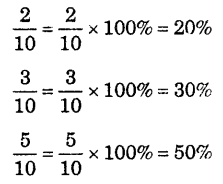(c) Total part of Ratio =1 + 4 = 5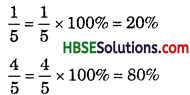(d) Total part of ratio = l + 2 + 5 = 8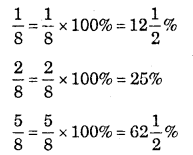Question 3.
The population of a city decreased from 25000 to 24500. Find the percent decrease.
Solution:
Percent decrease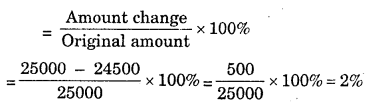∴ Percent decrease = 2%.

Question 4.
Arun bought a car for Rs. 3,50,000. The next year, the price went upto Rs. 3,70,000. What was the percent increase ?
Solution:
Percent increase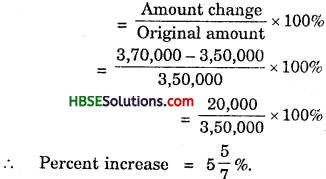Question 5.
I buy a T.V. for Rs. 10,000 and sell it at a profit of 20%. What do I get for it ?
Solution:
C.P. = Rs. 10,000, S.P. = ?
Profit = 20%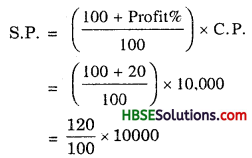∴ S.P. = Rs. 12000
Profit = S.P. – C.P.
= 12000 -10000
∴ Profit = Rs. 2000

Question 6.
Juhi sells a washing machine for Rs. 13,500. She loses 20% in the bargain. What was the price at which she bought it ? Solution:
S.P. = Rs. 13,500, loss = 20%,
C.P. = ?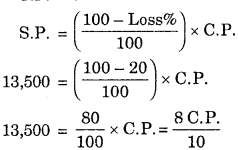or 8 C.P. = 13500 x 10
∴ C.P = $$\frac{135000}{8}$$ = Rs. 16875.Question 7.
(i) Chalk contains calcium, carbon and oxygen in the ratio 10 : 3 : 12. Find percent of carbon in chalk.
(ii) If in a stick of chalk, carbon is 3g, what is the weight of the chalk stick ?
Solution:
(i) Calcium : Carbon : Oxygen
= 10:3 : 12
Total part of Ratio = 10 + 3 + 12 = 25
Percent of calcium in chalk = $$\frac{10}{25}$$ x 100% = 40%
Percent of carbon in chalk = $$\frac{3}{25}$$ x 100% = 12%
Percent of oxygen in chalk = $$\frac{12}{25}$$ x 100% = 48%

(ii) Let total weight of carbon = x
According to question, 12% of x = 3 gm
or, $$\frac{12}{100}$$ x x = 3
x = $$\frac{3 x 100}{100}$$ = 25
Hence total weight of carbon = 25 gm.

Question 8.
Amina buys a book for Rs. 275 and sells it at a loss of 15%. IIow much does she sell it for ?
Solution:
C.P. = Rs. 275, S.P. = ?
Loss = 15%
S.P = $$\left(\frac{100-\operatorname{Loss} \%}{100}\right)$$ x 275 = Rs. 233.75

Question 9.
Find the amount to be paid at end of 3 years in each case :
(a) Principal = Rs. 1200 at 12% p.a.
(b) Principal = Rs. 7500 at 5%p.a.
Solution:
(a) S.I = $$\frac{\mathrm{PRT}}{100}$$
= $$\frac{1200 \times 12 \times 3}{100}$$ = Rs. 432
A = P + I = 1200 + 432
= Rs. 1632

(b) S.I. = $$\frac{7500 \times 5 \times 3}{100}$$ = Rs. 1125
A = P + I = 7500 + 1125
= Rs. 8625.Question 10.
What is the rate of interest which gives an interest of Rs. 280 on a sum of Rs. 56000 for 2 years ?
Solution:
P =Rs. 56000,
S.I. = Rs. 280, T = 2 years,
R =?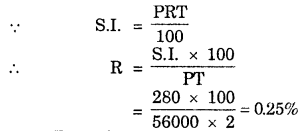.’. Rate of interest = 0.25%.

Question 11.
If Meena gives an interest of Rs. 45 for the one year at 9% rate p.a. What is sum she has borrowed ?
Solution:
S.I. = Rs. 45, R = 9%,
T = 1 year, P = ?
P = $$\frac{\text { S.I. } \times 100}{R \times T}=\frac{45 \times 100}{9 \times 1}$$
.’. Sum = Rs. 500.